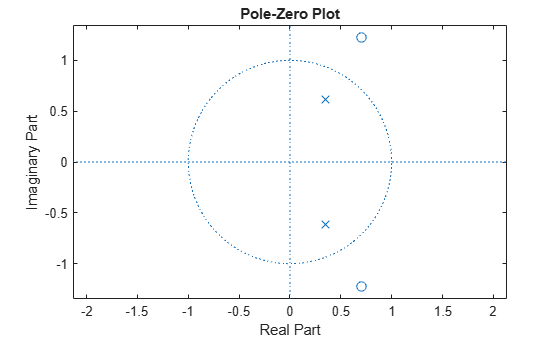# ismaxphase

Verify that discrete-time filter System object is maximum phase

## Syntax

``flag = ismaxphase(sysobj)``
``flag = ismaxphase(sysobj,tol)``
``flag = ismaxphase(___,'Arithmetic',arithType)``

## Description

example

````flag = ismaxphase(sysobj)` returns `true` if the filter System object™ has maximum phase.```
````flag = ismaxphase(sysobj,tol)` uses the tolerance `tol` to determine when two numbers are close enough to be considered equal. If not specified, `tol` defaults to `eps^(2/3)`.```
````flag = ismaxphase(___,'Arithmetic',arithType)` analyzes the filter System object based on the arithmetic specified in the `arithType` input using either of the previous syntaxes.For more input options, see `ismaxphase` in Signal Processing Toolbox™.```

## Examples

collapse all

Design an allpass filter and determine if the filter has maximum phase.

Using the `dsp.AllpassFilter` System object™, design an allpass filter that uses the minimum multiplier structure.

`a = dsp.AllpassFilter`
```a = dsp.AllpassFilter with properties: Structure: 'Minimum multiplier' AllpassCoefficients: [-0.7071 0.5000] TrailingFirstOrderSection: false ```

Using the `ismaxphase` function, determine if the filter has maximum phase.

`ismaxphase(a)`
```ans = logical 1 ```

Verify the location of poles and zeros of the filter transfer function on the z-plane. By definition, the zeros of the maximum phase filter must be outside the unit circle.

`zplane(a)`## Input Arguments

collapse all

Tolerance value to determine when two numbers are close enough to be considered equal, specified as a positive scalar. If not specified, `tol` defaults to `eps^(2/3)`.

Arithmetic used in the filter analysis, specified as `'double'`, `'single'`, or `'Fixed'`. When the arithmetic input is not specified and the filter System object is unlocked, the analysis tool assumes a double-precision filter. When the arithmetic input is not specified and the System object is locked, the function performs the analysis based on the data type of the locked input.

The `'Fixed'` value applies to filter System objects with fixed-point properties only.

When the `'Arithmetic'` input argument is specified as `'Fixed'` and the filter object has the data type of the coefficients set to `'Same word length as input'`, the arithmetic analysis depends on whether the System object is unlocked or locked.

• unlocked –– The analysis object function cannot determine the coefficients data type. The function assumes that the coefficients data type is signed, has a 16-bit word length, and is auto scaled. The function performs fixed-point analysis based on this assumption.

• locked –– When the input data type is `'double'` or `'single'`, the analysis object function cannot determine the coefficients data type. The function assumes that the data type of the coefficients is signed, has a 16-bit word length, and is auto scaled. The function performs fixed-point analysis based on this assumption.

To check if the System object is locked or unlocked, use the `isLocked` function.

When the arithmetic input is specified as `'Fixed'` and the filter object has the data type of the coefficients set to a custom numeric type, the object function performs fixed-point analysis based on the custom numeric data type.

## Output Arguments

collapse all

Flag to determine if the filter has maximum phase, returned as a logical:

• `1` –– Filter has maximum phase.

• `0` –– Filter has non maximum phase.

Data Types: `logical`

collapse all

### Maximum Phase Filters

A causal and stable discrete-time system is said to be strictly maximum-phase when all its zeros are outside the unit circle. A causal and stable LTI system is a maximum-phase system if its inverse is causal and unstable.

Such a system is called a maximum-phase system because it has the maximum group delay (`grpdelay`) of the set of systems that have the same magnitude response.